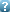VII-th International Conference "SOLITONS, COLLAPSES AND TURBULENCE: Achievements, Developments and Perspectives" (SCT-14) in honor of Vladimir Zakharov's 75th birthday August, 04-08, 2014 Chernogolovka, RussiaNonlinear dynamics of cosmological scalar fields with singular potentials
Date/Time: 16:30 05-Aug-2014
Abstract:
\begin{center}
{\Large Nonlinear dynamics of cosmological scalar fields}

{\Large with singular potentials}

\bigskip

V.A. Koutvitsky and \underline{E.M. Maslov}

\textit{IZMIRAN, Russia}
\end{center}

We investigate the dynamics of the inflaton scalar field $\phi (t,\mathbf{r}% )$ governed by the nonlinear Klein-Gordon equation in the
Friedmann-Robertson-Walker Universe,%
\begin{equation*}
\phi _{tt}+3H\phi _{t}-a^{-2}\Delta \phi +U^{\prime }(\phi )=0,
\end{equation*}%
where $a(t)$ is the scale factor, $H=a_{t}/a$ is the Hubble parameter. We
consider the potentials having singularity at their minimum, $\left| U^{\prime \prime }(\phi )\right| \rightarrow \infty \;(\phi \rightarrow 0)$.

First, the rapid oscillations of the homogeneous background $\phi (t)$ near
the minimum are studied. These damped oscillations determine growth of the
scale factor $a(t)$ through the Friedmann equations with the effective
pressure $p$ and energy density $\rho$ . To describe the oscillations we
make the transformation $(\phi ,\phi _{t})\rightarrow (\rho ,\theta )$,
representing the field as $\phi (t)=\varphi (\rho ,\theta )$, where the
function $\varphi (\rho ,\theta )$ is given by a quadrature, $\rho$ and $% \theta$ are slow and fast variables. Equations governing the evolution of
these variables are obtained in the Van der Pol approximation.

As examples, we consider two potentials with logarithmic and fractional
power singularities. For these potentials we calculate the equation of state
parameter $w=p/\rho$ and show that in some range of $\rho$ it lies in the
interval $-1<w<-1/3$, providing the accelerated expansion of the Universe.

Then we examine the resonant amplification of the field fluctuations $\delta \phi (t,\mathbf{r})$ on the oscillating background $\phi (t)$. The Fourier $k$-modes of $\delta \phi$ satisfy the singular Hill equation with slowly
varying parameters $\rho$ and $k/a$. Using the stability-instability chart,
calculated by the generalized Lindemann - Stieltjes method [2,3], we argue
that the fluctuations can be significantly amplified when the representative
trajectories of the parameters cross the resonance zones. At the nonlinear
stage these fluctuations can transform into well localized oscillating
lumps, the pulsons.

\bigskip

\textbf{References}

\bigskip

 M.S.~Turner, Phys. Rev.~D, \textbf{28}, 1243 (1983).

 V.A.~Koutvitsky and E.M. Maslov, J. Math. Phys., \textbf{47}, 022302
(2006).

  В.А. Кутвицкий, Е.М. Маслов, Проблемы мат. анализа (2014)
(в печати).

\end{document}

Attachments:

Authors
Maslov Eugene Michailovich(Presenter)